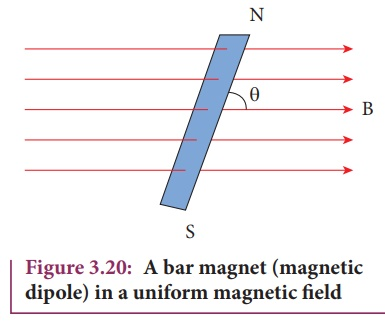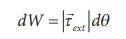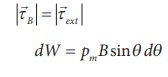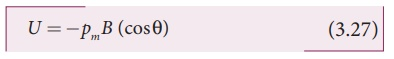Home | | Physics 12th Std | Potential energy of a bar magnet in a uniform magnetic field

# Potential energy of a bar magnet in a uniform magnetic field

Physics : Magnetism and Magnetic Effects of Electric Current: Potential energy of a bar magnet in a uniform magnetic field and with Solved Example Problems

Potential energy of a bar magnet in a uniform magnetic fieldWhen a bar magnet (magnetic dipole) of dipole momentm is held at an angle θ with the direction of a uniform magnetic field, as shown in Figure 3.20 the magnitude of the torque acting on the dipole isIf the dipole is rotated through a very small angular displacement dθ against the torque τB at constant angular velocity, then the work done by external torque () for this small angular displacement is given byThe bar magnet has to be moved at constant angular velocity, which implies thatTotal work done in rotating the dipole from θʹ to θ isThis work done is stored as potential energy in bar magnet at an angle θ when it is rotated from θʹ to θ and it can be written asIn fact, the equation (3.26) gives the difference in potential energy between the angular positions θʹ and θ. We can choose the reference point θʹ = 90o, so that second term in the equation becomes zero and the equation (3.26) can be written asThe potential energy stored in a bar magnet in a uniform magnetic field is given byCase 1

(i) If θ = 0º, then

= − pm B (cos0 º ) = − pm B

(ii) If θ = 180 º, then

U= − pm B (cos180 º) = pm B

We can infer from the above two results, the potential energy of the bar magnet is minimum when it is aligned along the external magnetic field and maximum when the bar magnet is aligned anti-parallel to external magnetic field.

EXAMPLE 3.8

Consider a magnetic dipole which on switching ON external magnetic field orient only in two possible ways i.e., one along the direction of the magnetic field (parallel to the field) and another anti-parallel to magnetic field. Compute the energy for the possible orientation. Sketch the graph.

Solution

Letm  be the dipole and before switching ON the external magnetic field, there is no orientation. Therefore, the energy U = 0.

As soon as external magnetic field is switched ON, the magnetic dipole orient parallel (θ = 0º) to the magnetic field with energy,

Uparallel = Uminimum = − pm B cos0

Uparallel = − pm B

since cos 0º = 1

Otherwise, the magnetic dipole orients anti-parallel (θ = 180º) to the magnetic field with energy,

Uanti− parallel = U maximum =− pmB cos180

anti−parallel = pmB

since cos 180º = -1Study Material, Lecturing Notes, Assignment, Reference, Wiki description explanation, brief detail
12th Physics : Magnetism and Magnetic Effects of Electric Current : Potential energy of a bar magnet in a uniform magnetic field |